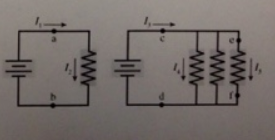# Problem: The figure shows two circuits. The two batteries are identical and the four resistors all have exactly the same resistance. Rank in order the five currents, I1 to I2.

###### FREE Expert Solution

In the first circuit, I1 = I2 because there is only one resistor as the component in the circuit.

For the second circuit, I3 = I1 = I2

86% (140 ratings)###### Problem Details

The figure shows two circuits. The two batteries are identical and the four resistors all have exactly the same resistance. Rank in order the five currents, Ito I2